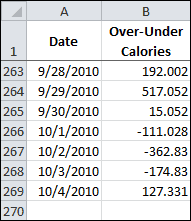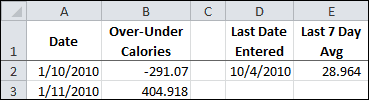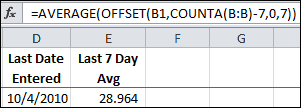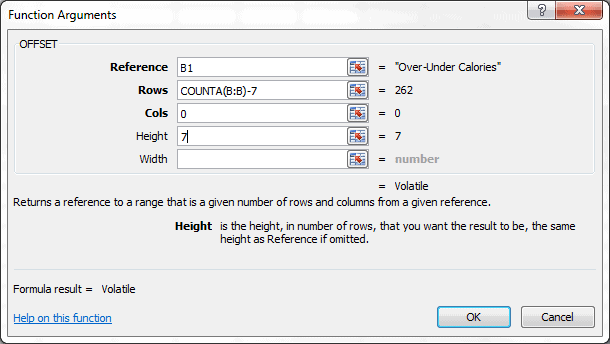The OFFSET Function – Last 7 Data Points

In this example I’m going to use the OFFSET Function to return a range reference to the last 7 cells in a column. Of course, it will be inside the AVERAGE Function so I can see an average for the last week.

Sound complicated? Not really, it’s just an extension of something I did on a previous post for the OFFSET Function.

The example data is a spreadsheet I use to log my over-under calories to goal each day. This data is downloaded periodically and currently the data goes to row 269, as you can see below.I use the OFFSET Function at the top of the worksheet to inform me of the Last 7 Day Average for the Over-Under Calories data, as shown below.Cell E2 has the following formula.

=AVERAGE(OFFSET(B1,COUNTA(B:B)-7,0,7)

The AVERAGE Function is merely taking the range reference provided by the OFFSET function to return an average. We’ll focus on the OFFSET Function and start with the arguments.

The OFFSET Function Arguments

The OFFSET Function has three required arguments — reference, rows, cols — and two optional arguments — height, width.

OFFSET(reference, rows, cols, [height], [width])

Reference is the anchor point, or ground zero. Everything starts here.

Rows is the number of rows from the anchor point. Or the row offset.  A positive number means down from the anchor point and a negative number means up from the anchor point.

Cols is the number of columns from the anchor point, or the column offset. A positive number means to the right of the anchor point and a negative number means to the left.

Height is the number of rows you want for the height of the range; a positive number, and if omitted is the same height as the reference.

Width is the number of columns you want for the width of the range; a positive number, and if omitted is the same width as the reference.

The OFFSET Function and the Last 7 Data Points

It’s useful knowing the 7-day average for over-under calories to goal. The OFFSET Function can give me this if I use it inside the AVERAGE function.For the OFFSET Function, the first argument reference is B1. That also means row 1 and column B when you break it down.

The second argument rows is COUNTA(B:B) -7, which computes to 269 – 7 = 262. The function COUNTA(B:B) is counting all non-blank cells in column B. Note: It’s important to remember that if any blank cells are in column B, the COUNTA Function won’t provide the right number to the rows argument.

Using 262 as the rows number, counting down from row 1 (the reference row) will plant you squarely in row 263, as the first row is not counted.

The third argument cols is zero. We’re not offsetting left or right.

The fourth argument height is 7. From the second argument we have row 263. Counting down 7 rows gives us the range reference B263:B269.

The fifth argument width, is omitted because we only want one column for the range.The OFFSET function returns the reference B263:B269, and that leaves the rest of the formula as AVERAGE(B263:B269) for this example, which results in the answer 28.964.

The formula in this example will always give and average for the last 7 data points in column B, as long as there are no blank cells.8 thoughts on “The OFFSET Function – Last 7 Data Points”

1.James

Pardon me, but the row argument wouldn’t be: “COUNTA(B:B) -8”, since it is 1 for the header plus 7 days?

1.Gregory

The header row isn’t counted in the row argument because it’s the reference row. Even though COUNTA will count the header row, the header row is the reference and considered row zero (0) when offsetting.

2.Jon Peltier

OFFSET(B1,COUNTA(B:B)-7,0,7)
is
OFFSET(B1,COUNTA(B:B),0,-7)
It’s slightly shorter, and it’s less complicated (in my mind anyway) to find the end of the range easily and work backward than to find the beginning of the range with more difficulty and work forward. I find the risk of an off-by-one error is reduced.

1.Gregory

When I put your formula OFFSET(B1,COUNTA(B:B),0,-7) inside the COUNTA Function there are 6 cells returned. When I do the same with OFFSET(B1,COUNTA(B:B)-7,0,7) I get 7 cells returned, which is what I was looking for – the last 7 Data points.

Your formula includes 7 cells, but one of those is the blank cell after the end of the range. When you offset from row 1 by the total row count, row 1 is considered zero in counting down, so you end up in the row after the end of the range. Then counting back up 7 rows gives the 7 rows, but one of them is blank.

An alternative formula would be OFFSET(B1,COUNTA(B:B)-1,0,-7) that would return the last 7 rows of the range.

1.Jon Peltier

Gregory –

I didn’t analyze the exact arguments based on your example, I just copied and pasted to give an example. I tend to use COUNT to count the number of values as opposed to the number of values plus labels. What you’ve noted is the off-by-one difference of using COUNT and COUNTA when the range contains a non-numeric header row. The formula I should have entered is
OFFSET(B1,COUNT(B:B),0,-7)

1.Gregory

Jon –
I hadn’t consider using the COUNT function. Now the second argument will match the number of offset rows to the end of the range. Very nice. Many thanks for pointing that out.